#### Thank you for registering.

One of our academic counsellors will contact you within 1 working day.

Please check your email for login details.

Click to Chat

1800-1023-196

+91-120-4616500

CART 0

• 0
MY CART (5)

Use Coupon: CART20 and get 20% off on all online Study Material

ITEM
DETAILS
MRP
DISCOUNT
FINAL PRICE
Total Price: Rs.

There are no items in this cart.
Continue Shopping• Complete JEE Main/Advanced Course and Test Series
• Offered Price: Rs. 15,900
• View Details

# Chapter 14: Coordinate Geometry Exercise – 14.2

### Question: 1

Find the distance between the following pair of points:

(i) (- 6, 7) and (-1, -5)

(ii) (a + b, b + c) and (a -b, c - b)

(iii) (a sin α, - b cos α) and (- a cos α, b sin α)

(iv) (a, 0) and (0, b)

### Solution:

(i) We have P (- 6, 7) and Q (- 1, - 5)

Here,

x1 = - 6, y1 = 7 and

x2 = -1, Y2 = - 5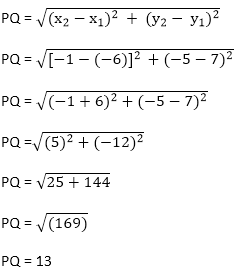(ii) We have P (a + b, b + c) and Q (a - b, c - b) here,

x1 = a + b, y= b + c and x2 = a - b, Y2 = c - b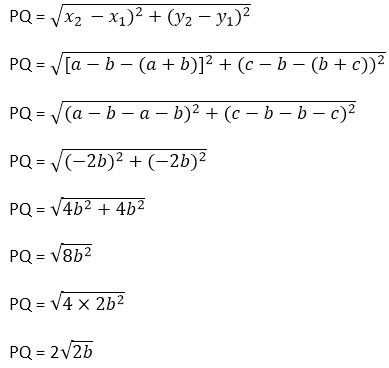(iii) We have P(a sinα, – b cos α) and Q(-a cos α, b sin α) here

x1 = a sin α, y1 = - b cos α and

x2 – a cos α, y2 = b sin α(iv) We have P(a, 0) and Q (0, b)

Here,

x1 = a,y1 = 0, x2 = 0, y2 = b,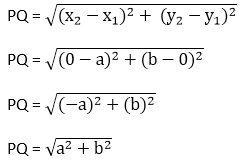### Question: 2

Find the value of a when the distance between the points (3, a) and (4, 1) is √10.

### Solution:

We have P (3, a) and Q(4, 1)

Here,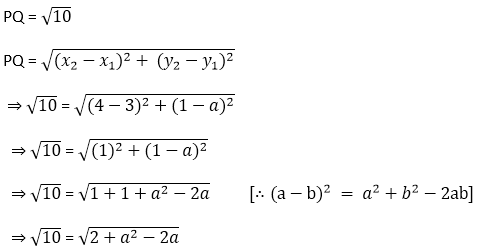Squaring both sides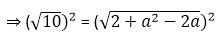⇒ 10 = 2 + a2 – 2a

⇒ a2 – 2a + 2 – 10 = 0

⇒ a2 – 2a – 8 = 0

Splitting the middle team

⇒ a2 – 4a + 2a - 8 = 0

⇒ a(a - 4) + 2(a - 4) = 0

⇒ (a - 4) (a + 2) = 0

⇒ a = 4, a = - 2

### Question: 3

If the points (2, 1) and (1, -2) are equidistant from the point (x, y) from (-3, 0) as well as from (3, 0) are 4.

### Solution:

We have P(2, 1) and Q(1,- 2) and R(X, Y)

Also, PR = QR∴ PR = QR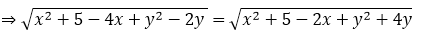⇒ x2 + 5 - 4x + y2 –2y = x2 + 5 - 2x + y2 + 4y

⇒ x2 + 5 - 4x + y2 – 2y = x2 + 5 - 2x + y2 + 4y

⇒ - 4x + 2x - 2y - 4y = 0

⇒ - 2x – 6y = 0

⇒ - 2(x + 3y) = 0

⇒ -2(x + 3y) = 0

⇒ x + 3y = 0/-2

⇒ x + 3y = 0

Hence Proved.

### Question: 4

Find the value of x, y if the distances of the point (x, y) from (- 3, 0) as well as from (3, 0) are 4.

### Solution:

We have P(x, y), Q( -3, 0) and R(3, 0)Squaring both sides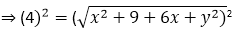⇒ 16 = x2 + 9 + 6x + y2

⇒ x2 + y2 = 7 - 6x                            …… (1)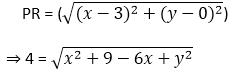Squaring both sides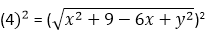⇒16 = x2 + 9 – 6x + y2

⇒ x2 + y2 = 16 – 9 + 6x

⇒ x2+ y2 = 7 + 6x                   …. (2)

Equating (1) and (2)

7 - 6x = 7 + 6x

⇒ 7 – 7 = 6x + 6x

⇒ 0 = 12x

⇒ x = 12

Substituting the value of x = 0 in (2)

x2 + y2 = 7+ 6x

0 + y2 = 7 + 6 × 0

Y2 = 7

Y = + 7## Complete JEE Main/Advanced Course and Test Series

OFFERED PRICE: Rs. 15,900
View Details Xpress Buy

### Course Features

• 731 Video Lectures
• Revision Notes
• Test paper with Video Solution
• Mind Map
• Study Planner
• NCERT Solutions
• Discussion Forum
• Previous Year Papers

### Course Features

• 728 Video Lectures
• Revision Notes
• Previous Year Papers
• Mind Map
• Study Planner
• NCERT Solutions
• Discussion Forum
• Test paper with Video Solution